Courses

# Engineering Mathematics 3

## 10 Questions MCQ Test RRB JE for Computer Science Engineering | Engineering Mathematics 3

Description
This mock test of Engineering Mathematics 3 for Computer Science Engineering (CSE) helps you for every Computer Science Engineering (CSE) entrance exam. This contains 10 Multiple Choice Questions for Computer Science Engineering (CSE) Engineering Mathematics 3 (mcq) to study with solutions a complete question bank. The solved questions answers in this Engineering Mathematics 3 quiz give you a good mix of easy questions and tough questions. Computer Science Engineering (CSE) students definitely take this Engineering Mathematics 3 exercise for a better result in the exam. You can find other Engineering Mathematics 3 extra questions, long questions & short questions for Computer Science Engineering (CSE) on EduRev as well by searching above.
QUESTION: 1

### The matrix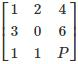has one eigenvalue equal to 3. The sum of the other two eigenvalues is

Solution:

Sum of the eigen values of matrix is = Sum of diagonal values present in the matrix

∴ 1 + 0 + P = 3 + λ+ λ3

⇒ P + 1 = 3 + λ+ λ3

⇒ λ+ λ= P + 1 – 3 = P – 2

QUESTION: 2

### What is the determinant of matrix X if 4 and (2 + 7i) are the eigenvalues of X where i = √−1?

Solution:

Two eigen value of X is 4 and (2 + 7i)

∴ (2 - 7i) (conjugate roots) must be the third root

Determinant of P = product of eigenvalues

Δ = 4 × (2 + 7i) × (2 - 7i)

Δ = 212

QUESTION: 3

### In the given matrix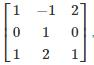one of the eigenvalues is 1. The eigenvectors corresponding to the eigenvalue 1 are

Solution:

For a given matrix A if V is the eigen vector corresponding to the eigen value λ, then:

AV = λV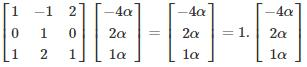∴ {α (−4, 2, 1) |α ≠ 0, αϵR} are the corresponding eigenvectors.

QUESTION: 4

Which of the below-given statements is/are true?

I. The eigenvalue of the lower triangular matrix is just the diagonal elements of the matrix.

II. The product of the eigenvalue of a matrix is equal to its trace.

III. If 1/λ is an eigenvalue of A’(inverse of A) then orthogonal of A also have 1/λ as its eigenvalue.

Solution:
• The eigenvalue of the triangular (lower or upper) matrix are just the diagonal elements of matrix.
• The product of the eigenvalue of a matrix is equal to its determinants.
• If λ is the eigenvalue of matrix, then 1/λ is the eigenvalue of its inverse since orthogonal is equal to inverse matrix then it has 1/λ as its eigenvalue

​Example: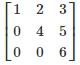Eigenvalues are 1, 4 and 6 (diagonal elements)

Product of eigen value = determinants = 1 × 4 × 6 = 24

Orthogonal matrix and Inverse of given matrix have eigenvalues: 1,1/4 and 1/6

QUESTION: 5

Consider the following 2 × 2 matrix A where two elements are unknown and are marked by a and b. The eigenvalues of this matrix are - 1 and 7. What are the values of a and b?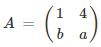Solution:

W.K.T.∑λ= ∑aii
∴ - 1 + 7 = 1 + a ⇒ a = 5

Also π λi = |A|

∴ - 1 × 7 = a – 4b

- 7 = 5 – 4b ⇒ b = 3

QUESTION: 6

Let A be the 2 X 2 matrix with elements a11 = 2, a12 = 3, a21 = 1 and a22 = 4 then the eigenvalues of the Matrix are A5?

Solution:

Given Matrix: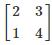Characteristic Equation: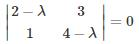(2−λ) (4−λ) −3 = 0

λ2 − 6λ + 5 = 0

λ = 5 or λ = 1

*Answer can only contain numeric values
QUESTION: 7

The latent values of the matrix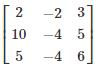are 1, 1, 2 and the number of linearly independent latent vectors for the repeated root 1 is –

Solution:

Eigen vectors are also called invariant vectors, characteristic vectors, or latent vectors. Eigenvalues are also called characteristic roots or latent roots.

(A - λI)X = 0

Given λ = 1

⇒ (A - I) X = 0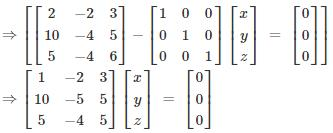⇒ x = 2y + 3x = 0, 10x – 5y + 5z = 0, 5x – 4y + 5z = 0

By solving above equations, we get x =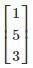i.e. one linearly independent latent vector.

*Answer can only contain numeric values
QUESTION: 8

Consider a Matrix M = uTvT where u =  (112) and v =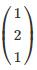also uT denotes the transpose of matrix u. Find the largest eigenvalue of M?

Solution: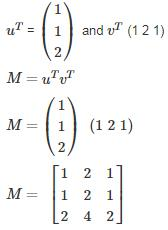Characteristic equation is given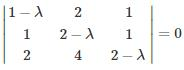λ3 − 5λ2 = 0

λ = 0 or λ = 5

Therefore largest value is 5.

QUESTION: 9

What is the absolute difference of the eigenvalues for the matrix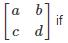ad – bc = 6 and a + d = 7?

Solution:

Let λ1 and λ2 be the two eigen values

Product of eigen value is equal to determinant of matrix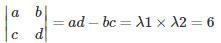Sum of eigen value is equal to its trace

a + d = λ1 + λ2 = 7

λ1 × (7 − λ1) = 6

λ12 − 7λ + 6 = 0

λ1 = 6 or λ2 = 1

|λ1 − λ2| = 5

QUESTION: 10

Consider the matrix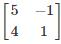which one of the following statements is TRUE for the eigenvalues and eigenvectors of the matrix?

Solution: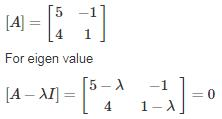(5 - λ) (1 - λ) + 4 = 0

5 – 5λ - λ + λ2 + 4 = 0

λ2 - 6λ + 9 = 0

(λ - 3)2 = 0

λ = 3

for eigen vector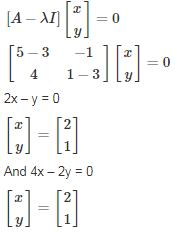So, only one independent eigen vector.